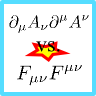An Alternative LagrangianUsing the useful index notation of special relativity we will find that one may use different Lagrangians to describe relativistic electrodynamics. This problem will make you familiar with the basic rules needed for practical calculations.

Problem Statement

In some textbooks we find the relativistic Lagrangian of electrodynamics in the form$\begin{eqnarray*} \mathcal{L}_{1} = -\frac{1}{2\mu_{0}}\partial_{\mu}A_{\nu}\partial^{\mu}A^{\nu}-J_{\mu}A^{\mu}\ .\end{eqnarray*}$Usually, however, it is given as$\begin{eqnarray*} \mathcal{L}_{2} = -\frac{1}{4\mu_{0}}F_{\mu\nu}F^{\mu\nu}-J_{\mu}A^{\mu}\ .\end{eqnarray*}$Calculate the equations of motion for the first term to find the hidden assumption for which both Lagrangians are equivalent.